# 目录：

1、基本定义

2、散列表思想

1、定义

2、散列函数设计的基本要求

3、如何设计散列函数

1、开放寻址法

2、链表法

3、如何选择散列冲突解决的方法

1、Java中的HashMap

1、Word文档中单词拼写检查功能

2、假设我们有10万条URL访问日志，如何按照访问次数给URL排序？

3、有两个字符串数组，每个数组大约有10万条字符串，如何快速找出两个数组中相同的字符串？

# 一、散列表基本概念

### 2、散列表思想

（1）使用散列函数将给定键转化为一个“数组的索引”，理想情况下，不同的key会被转化为不同的索引，但是在实际情况中，我们会遇到不同的键转化为相同索引的情况，这种情况叫做散列冲突/碰撞，后文中会详细讲解；

（2）得到了索引后，我们就可以像访问数组一样，通过这个索引访问到相应的键值对。

# 二、散列函数

### 2、散列函数设计的基本要求

（1）散列函数计算得到的散列值是一个非负整数;

（2）如果key1  =  key2，那么hash(key1)  ==  hash(key2);

（3）如果key1  !=  key2，那么hash(key1)  !=  hash(key2)。

### 3、如何设计散列函数

（1）散列函数的设计不能太复杂，过于复杂的散列函数，势必会消耗很多计算时间，也会间接的影响到散列表的性能；

（2）散列函数生成的值要尽可能随机且均匀分布，这样才能避免或者最小化散列冲突，而且即便出现冲突，散列到每个槽里的数据也会比较平均，不会再出现某个槽里数据特别多的情况。

<1> 直接寻址法：

<2> 数字分析法：

<3> 平方取中法：

KEYA11050201122157778355001778
KYAB11250102126564795010404795
AKEY01110525001233265775625265
BKEY02110525004454315775625315

<4> 折叠法：

<5>随机数法：

<6>除留取余法：

【说明：上诉6种方法出自于：https://blog.csdn.net/xiaoxik/article/details/74926090

# 三、散列冲突

【ps:此段落内容摘抄自极客时间的《数据结构与算法之美》专栏】

### 1、开放寻址法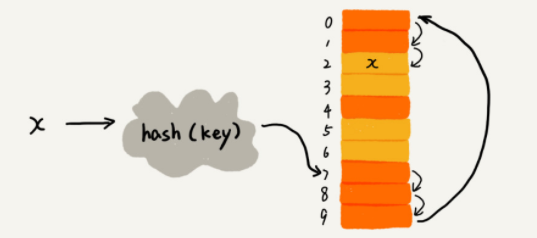图1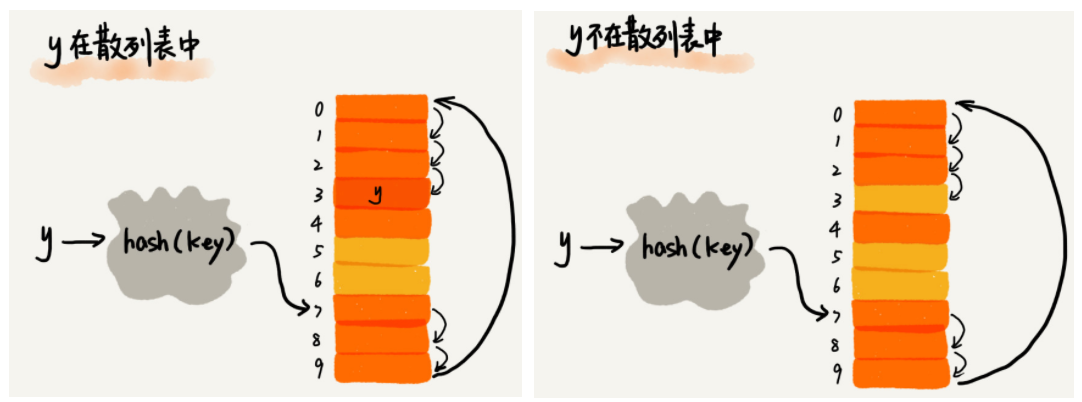图2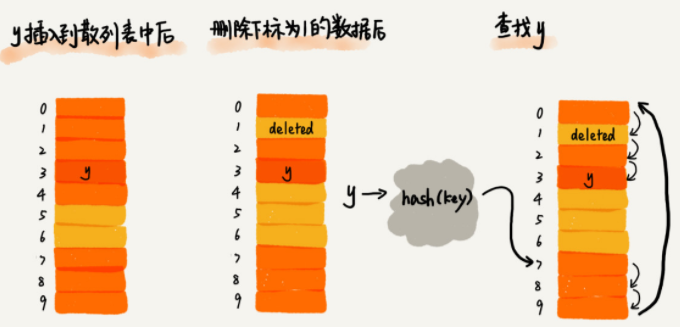图3

public class LinearProbingHashMap<K, V> {

private int num;       // 散列表中键值对数目
private int capacity;  // 散列表的大小
private K[] keys;      // 散列表中的键
private V[] values;    // 散列表中的值

public LinearProbingHashMap(int capacity){
keys = (K[]) new Object[capacity];
values = (V[]) new Object[capacity];
this.capacity = capacity;
}

// 散列函数
private int hash(K key){
return (key.hashCode() & 0x7fffffff) % capacity;
}

// get()方法
public V get(K key){
int index = hash(key);
while(keys[index] != null && !keys.equals(keys[index])){
index = (index + 1) % capacity;
}
// 若给定key在散列表中存在会返回相应value，否则这里返回的是null
return values[index];
}

// put()方法
public void put(K key, V value){
if(num >= capacity / 2){
resize(2 * capacity);
}

int index = hash(key);
while(keys[index] != null && !keys.equals(keys[index])){
index = (index + 1) % capacity;
}

if(keys[index] == null){
keys[index] = key;
values[index] = value;
return;
}

values[index] = value;
num++;
}

// 删除操作
public void delete(K key){

if((keys.toString()).contains((CharSequence) key)){
return;
}

int index = hash(key);

while(!key.equals(keys[index])){
index = (index + 1) % capacity;
}

keys[index] = null;
values[index] = null;
index = (index + 1) % capacity;
while(keys[index] != null){
K keyToRedo = keys[index];
V valueToRedo = values[index];
keys[index] = null;
values[index] = null;
num--;
put(keyToRedo, valueToRedo);
index = (index + 1) % capacity;
}
num--;
if(num > 0 && num == capacity / 8){
resize(capacity / 8);
}
}

// 动态扩容
private void resize(int newCapacity) {
LinearProbingHashMap<K, V> hashmap = new LinearProbingHashMap<>(newCapacity);
for (int i = 0; i < capacity; i++) {
if (keys[i] != null) {
hashmap.put(keys[i], values[i]);
}
}
keys = hashmap.keys;
values = hashmap.values;
capacity = hashmap.capacity;
}

}


### 2、链表法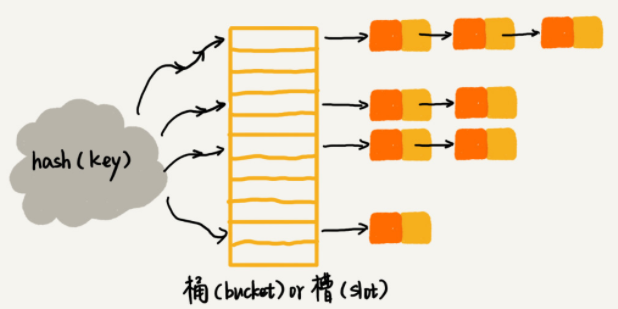图4

class ChainingHashSet<K, V> {

private int num;       			// 当前散列表中的键值对总数
private int capacity;  			// 散列表的大小
private SeqSearchST<K, V>[] st;   // 链表对象数组

// 构造函数
public ChainingHashSet(int initialCapacity){
capacity = initialCapacity;
st = (SeqSearchST<K, V>[]) new Object[capacity];
for(int i = 0; i < capacity; i++){
st[i] = new SeqSearchST<>();
}
}

// hash()方法
private int hash(K key){
return (key.hashCode() & 0x7fffffff) % capacity;
}

public V get(K key){
return st[hash(key)].get(key);
}

public void put(K key, V value){
st[hash(key)].put(key, value);
}
}

// SeqSearchST基于链表的符号表实现
class SeqSearchST<K, V>{

private Node first;

// 结点类
private class Node{
K key;
V value;
Node next;

// 构造函数
public Node(K key, V val, Node next){
this.key = key;
this.value = val;
this.next = next;
}
}

// get()方法
public V get(K key) {
for(Node node = first; node != null; node = node.next){
if(key.equals(node.key)){
return node.value;
}
}
return null;
}

// put()方法
public void put(K key, V value) {
// 先查找表中是否已经存在相应的key
Node node;
for(node = first; node != null; node = node.next){
if(key.equals(key)){
node.value = value;  // 如果key存在，就把当前value插入node.next中
return;
}
}

// 表中不存在相应的key，直接插入表头
first = new Node(key, value, first);
}
}

（1）开放寻址法

（2）链表法

# 四、装载因子

1、装载因子越小，发生的可能性就越小；

2、装载因子越大，代表着表中已填入的元素越多，再填入元素时发生冲突的概率也就越大。那么在查找时，给定值需要比较的关键字的个数就越多。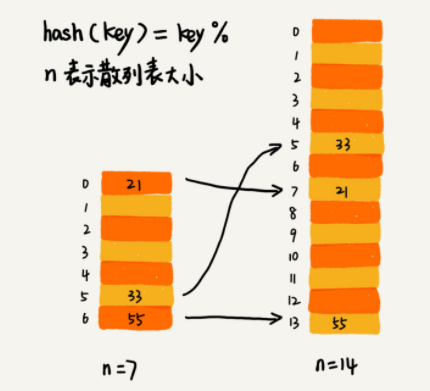图5

### 现在，我们需要考虑一个问题，如何避免上诉中的低效扩容？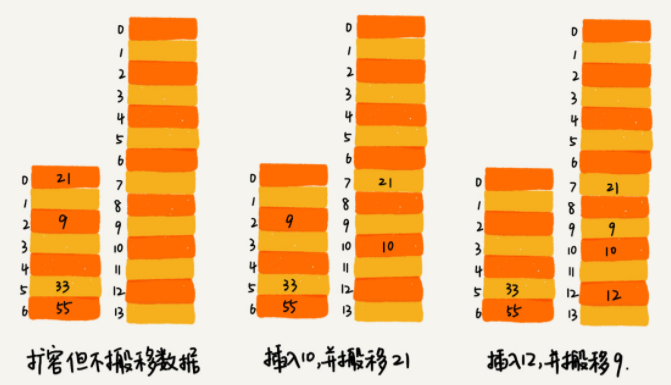图6

# 五、散列表应用场景

### 1、Java中的HashMap

（1）、初始大小：HashMap默认的大小是16，如果事先知道大概数据量有多少，可以通过修改默认初始大小，减少动态扩容的次数，这样会大大提高HashMap的性能。

（2）、装载因子和动态扩容：最大装载因子默认是0.75，当HashMap中元素个数超过0.75*capacity（capacity表示散列表的容量）的时候，就会启动自动扩容，每次扩容为原来的2倍；

（3）、散列冲突解决方法：HashMap底层采用链表法来解决冲突，即便负载因子和散列函数设计的再合理，也避免不了出现拉链过长的情况，一旦出现拉链过长，就会严重影响HashMap的性能。于是，在JDK1.8中，对HashMap做了进一步的优化，引入了红黑树。当链表长度大于8（默认长度）时，链表就会转化为红黑树。当红黑树的结点个数小于8时，有转化为链表。因为在数据量较小的时候，红黑树需要维护平衡，比起链表并没有太大的优势。

（4）、散列函数：源码如下：

int hash(Object key){
int h = key.hashCode();
return (h ^ (h >>> 16)) & (capacity - 1);  // capacity表示散列表的大小
}

public int hashCode() {
int var1 = this.hash;
if(var1 == 0 && this.value.length > 0) {
char[] var2 = this.value;
for(int var3 = 0; var3 < this.value.length; ++var3) {
var1 = 31 * var1 + var2[var3];
}
this.hash = var1;
}
return var1;
}


public class HashMap<K,V> extends AbstractMap<K,V> implements Map<K,V>, Cloneable, Serializable {

一、静态成员变量
// 默认的容量为16
static final int DEFAULT_INITIAL_CAPACITY = 16;

// 最大容量为2的30次方
static final int MAXIMUM_CAPACITY = 1 << 30;

// 默认的装载因子是0.75
static final float DEFAULT_LOAD_FACTOR = 0.75f;

// 默认的是Entry数组
transient Entry<K,V>[] table;

// table中实际的Entry数量
transient int size;

// size达到此门槛后，必须扩容table，值为：capacity * loadfactory = 16 * 0.75 = 12，也就意味着，存够12个数据时，table就要扩容一次
int threshold;

// 装载因子，值从一开始构造HashMap时就确定了，默认是0.75
final float loadFactor;

transient int modCount;

static final int ALTERNATIVE_HASHING_THRESHOLD_DEFAULT = Integer.MAX_VALUE;

private static class Holder { ...//省略}

......
......

二、构造函数
// 初始容量initialCapacity为16，装填因子loadFactor为0.75
public HashMap(int initialCapacity, float loadFactor) {
if (initialCapacity < 0)
throw new IllegalArgumentException("Illegal initial capacity: " +
initialCapacity);
if (initialCapacity > MAXIMUM_CAPACITY)
initialCapacity = MAXIMUM_CAPACITY;
if (loadFactor <= 0 || Float.isNaN(loadFactor))
throw new IllegalArgumentException("Illegal load factor: " +
loadFactor);

int capacity = 1;
while (capacity < initialCapacity)
capacity <<= 1;

this.loadFactor = loadFactor;
threshold = (int)Math.min(capacity * loadFactor, MAXIMUM_CAPACITY + 1);
table = new Entry[capacity];
useAltHashing = sun.misc.VM.isBooted() &&
(capacity >= Holder.ALTERNATIVE_HASHING_THRESHOLD);
init(); // init可以忽略，方法默认为空{}，当你需要集成HashMap实现自己的类型时可以重写此方法做一些事

}

 // 三、Map.Entry<K, V>,数组table实际存储的类型
static class Entry<K,V> implements Map.Entry<K,V> {
final K key;
V value;
Entry<K,V> next;
int hash;

// Entry的构造函数
Entry(int h, K k, V v, Entry<K,V> n) {
value = v;
next = n;    // 链表的下一个Entry
key = k;
hash = h;
}

// hashCode()方法
public final int hashCode() {
return (key==null   ? 0 : key.hashCode()) ^
(value==null ? 0 : value.hashCode());
}

// 存数据
public V put(K key, V value) {
if (key == null)
// HashMap允许key为null：在table中找到null key，然后设置Value，同时其hash为0；
return putForNullKey(value);

// a、计算key的hashCode
int hash = hash(key);

// b、根据hashCode计算index
int i = indexFor(hash, table.length);

// c、遍历index位置的Entry链表
for (Entry<K,V> e = table[i]; e != null; e = e.next) {
Object k;
if (e.hash == hash && ((k = e.key) == key || key.equals(k))) {

// hashCode和equals都相等则表明：本次put是覆盖操作，下面return了被覆盖的老value
V oldValue = e.value;
e.value = value;
e.recordAccess(this);
return oldValue;
}
}

modCount++;

// d、添加Entry，并解决冲突
// 如果需要增加table长度（size>threshold）就乘2增加，并重新计算每个元素在新table中的位置和转移
addEntry(hash, key, value, i);
return null;   // 增加成功最后返回null

}

a、为了防止低质量的hash函数，HashMap自己会再重新计算一遍key的hashCode

final int hash(Object k) {
int h = 0;
if (useAltHashing) {
// 字符串会被特殊处理，返回32bit的整数（就是int）
if (k instanceof String) {
return sun.misc.Hashing.stringHash32((String) k);
}
h = hashSeed;
}

// 将key的hashCode与h按位异或，最后赋值给h
h ^= k.hashCode();

h ^= (h >>> 20) ^ (h >>> 12);
return h ^ (h >>> 7) ^ (h >>> 4);
}

b、计算此hashCode该被存放入table的那个index

static int indexFor(int h, int length) {
// 与table的length - 1按位与，就能保证返回结果在0-length-1内
return h & (length-1);
}

c、解决冲突，链表法

void createEntry(int hash, K key, V value, int bucketIndex) {
Entry<K,V> e = table[bucketIndex];  // index的Entry拿出来
// put添加新元素是直接new Entry放在链头，如果有老的（有冲突）则将next设置为老的，如果没有正好设置next为null
table[bucketIndex] = new Entry<>(hash, key, value, e);
size++;
}

public V get(Object key) {
if (key == null)
return getForNullKey();
// 3 如果该index处Entry的key与此k相等，就返回value，否则继续查看该Entry的next
Entry<K,V> entry = getEntry(key);

return null == entry ? null : entry.getValue();
}

final Entry<K,V> getEntry(Object key) {
// 1 根据k使用hash(k)重新计算出hashCode
int hash = (key == null) ? 0 : hash(key);
// 2 根据indexFor计算出该k的index
for (Entry<K,V> e = table[indexFor(hash, table.length)];
e != null;
e = e.next) {
Object k;
if (e.hash == hash &&
((k = e.key) == key || (key != null && key.equals(k))))
return e;
}
return null;
}

这里从HashMap延申到Object对象【Object类API解读】：

public class Object {

// ...
//hashCode()方法
public native int hashCode();

// equals()方法
public boolean equals(Object obj) {
return (this == obj);
}

// ...
}

equals()方法上说明了：覆盖此方法时，通常有必要重写hashCode()方法，以维护equals的对象必须有相同的哈希码。

# 六、总结

1、散列表来源于数组，它借助散列函数对数组这种数据结构进行扩展，利用的是数组支持按照下标随机访问元素的特性；

2、散列表的两个核心问题是散列函数散列冲突的解决；

3、散列冲突有两种常用的解决办法：开放寻址法链表法；

4、散列函数设计的好坏决定了散列冲突的概率，也就是决定了散列表的性能；

5、如何根据装载因子进行动态扩容。

6、散列表的应用场景。

### 参考及推荐：

1、java中哈希表及其应用详解

2、Java数据结构与算法解析——散列表

3、散列表的基本原理与实现08-25
06-0322万+
05-2018万+
10-262030
06-151038
01-2389
04-2581
07-22173
10-309890
04-24931
10-23
10-171万+
01-27132
10-131万+
05-07743
08-31118
11-05280
©️2020 CSDN 皮肤主题: 大白 设计师:CSDN官方博客